# Mixed convection in complicated cavity with non-uniform heating on both sidewalls

Mixed convection in complicated cavity with non-uniform heating on both sidewalls

Said Mekroussi*Sahraoui Kherris Brahim Mebarki Ahmed Benchatti

Laboratoire de Technologies Industrielle, Université Ibn Khaldoun, Tiaret 14000, Algérie

Institut des Sciences et de la Technologie, Centre Universitaire El Wancharissi de Tissemsilt, Tissemsilt 38000, Algérie

Laboratoire d'énergétique en Zones Arides, Université Tahri Mohammed Béchar, B.P.417, Bechar08000, Algérie

Laboratoire de Mécanique, Université Amar Telidji Laghouat, B.P. 37G, Laghouat 03000, Algérie

Corresponding Author Email:
mekroussi_said@yahoo.fr
Page:
1023-1033
|
DOI:
https://doi.org/10.18280/ijht.350439
|
Accepted:
|
Published:
31 December 2019
| Citation

OPEN ACCESS

Abstract:

This paper deals with a numerical study of mixed convection flow and heat transfer inside lid-driven cavity.  The main purpose in this present study is to maximize the heat transfer. For that, a new lid-driven cavity with two wavy walls and non-uniform heating on both sidewalls has been proposed. The two horizontal walls are considered isolated, while two variable sinusoidal temperature distributions are imposed to the left and right vertical side walls with different amplitudes and phases. A finite volume method is used to solve numerically the non-dimensional governing equations. The tests were carried out for Richardson numbers which vary from 0.01 to 100, amplitude ratios from 0 to 1, and phase deviations from 0 to p, meanwhile the Prandtl number is fixed to Pr = 0.71. The distributions of streamlines, isotherms, and the variations of local and average Nusselt numbers with the variation of Richardson numbers, amplitude ratios and phase deviations are presented and compared with results given in the literature. The comparisons have showed a satisfactory agreement. Furthermore, the present study showed that the wavy walls with variable temperature have strong influence on enhancing heat transfer.

Keywords:

Mixed Convection, Lid-driven Cavity, Wavy Wall, Spatially Variable Temperature, Amplitude, Phase Deviation.

1. Introduction

The study of mixed convection heat transfer in lid-driven cavity has received a great deal of attention from researchers in recent years. This problem is commonly encountered in a variety of engineering applications. It is necessary to note that the mixed convection in a lid-driven cavity has received considerable attention in recent years. Thus, the present literature tries to review the previous studies. Iwatsu et al.  computationally investigated the flow of a viscous thermally stratified fluid in a square cavity. The flow was driven by both the top lid and buoyancy. They investigated the possibility of a resonance structure giving rise to the intensification of the flows in the interior and associated augmentation of convective heat transport. Later on, Iwatsu et al.  and Iwatsu and Hyun  respectively conducted two- and three-dimensional numerical simulation of mixed convection in a square cavity heated from the top moving wall. Mixed convection in enclosures of various boundary conditions has been studied by Gebhart et al.  and Hasanoui et al. . Lee and Chen  obtained finite element solutions of mixed convection in a bottom heated square cavity. Moraga and Lopez  performed a numerical analysis of three-dimensional model of mixed convection in an air-cooled cavity in order to compare the variations in different properties with the results of two-dimensional models. Wang and Chen  analyzed forced convection in a wavy-wall channel and demonstrated the effects of wavy geometry, Reynolds number and Prandtl number on the skin friction and Nusselt number. Al-Amiri et al.  investigated mixed convection heat transfer in lid-driven cavity with a sinusoidal wavy bottom surface. Zeghbid and Bessaih  studied numerically the mixed convection in lid-driven cavities filled with a nanofluid. Mixed convection of power-law fluids along a vertical wedge with convective boundary condition in a porous medium have been studied by Khan and Gorla . It is worth noting that most of the previous studies on square enclosures were concerned with natural convection. For example, Adjlout et al.  reported a numerical study of the effect of a hot wavy wall in an inclined differentially heated square cavity. Aich et al.  analyzed the natural convection in a prismatic enclosure, they indicated that the temperature decreases by increasing Rayleigh number. Numerical simulations of fluid flow and heat transfer in a four-sided lid-driven rectangular domain have been investigated by Ambethka and Kushawaha . Sharif  investigated laminar mixed convection processes in shallow two-dimensional rectangular cavities at three different Richardson numbers representing the dominating forced convection, mixed convection, and dominating natural convection using the Fluent commercial code. Boutara et al.  made a study on free convection enhancement within a nanofluid filled enclosure with square heaters. Kumar  studied numerically the natural convection in a porous enclosure with their vertical wavy surfaces under constant heat flux. The natural convection along a vertical wavy surface has been studied theoretically for the application of a cooling fin . Mekroussi et al.  numerically investigated the mixed convection in an inclined lid-driven cavity with a wavy wall. Recently, Lorenzini et al.  have investigated the constructal design of rectangular fin intruded into mixed convective lid-driven cavity flows. Messaoudi et al.  analyzed numerical study of mixed convection and flow pattern in various across-shape concave enclosures. Ambethka and Kumar  numerecally studied the fluid flow and heat transfer in a four-sided lid-driven rectangular domain.

Most studies devoted to cavities heated with a constant temperature or a constant heat flux on the wall, while in reality these thermal boundary conditions seldom exist, for example, the cooling of electronic components may be subjected to a non-uniform distribution of temperature. There is a large number of studies on both mixed and natural convection heat transfer in cavities with non-uniformly temperature distribution on their walls, but studies of a cavity with a wavy wall and non-uniformly temperature distribution are rare, and this is our concern of study in this paper. Oztop and Varol  performed a numerical study to obtain combined convection field in inclined porous lid-driven enclosures heated from one wall with a non-uniformly heater. Bae et al.  made a numerical analysis and experimental research of the natural convection in a rectangular enclosure with a heating source. Dalal and Das  investigated by the CFD method the inclined right wall wavy enclosure with spatially variable temperature boundary conditions. Deng and Chang  investigated the natural convection in a rectangular enclosure with sinusoidal temperature distributions on both side walls and have concluded that the natural convection heat transfer in enclosures with two sinusoidal temperature distributions on the side walls is superior to that with a single sinusoidal temperature profile on one side wall. Natarajan et al.  conducted a study using a penalty finite element analysis with bi-quadratic elements to investigate the effect of uniform and non-uniform heating of the bottom wall on natural convection flows in a trapezoidal cavity. Sivasankaran et al.  investigated natural convection in an inclined rectangular enclosure filled by the air with sinusoidal boundary condition. Very recently, mixed convection flow and heat transfer in a square lid-driven cavity filled with fluid saturated porous medium with sinusoidal temperature distribution on both side walls was studied by Sivasankaran et al. . Abbasian et al.  have numerically studied mixed convection flow in a lid-driven cavity with sinusoidal heating on sidewalls using nanofluid.

A thorough search in the literature suggests that little space has been devoted to the study of mixed convection in lid-driven cavity. In this study, a new lid-driven cavity with two wavy walls has been proposed. The horizontal cavity walls are insulated with movable top wall and the two vertical wavy walls have two spatially varying sinusoidal temperature distributions with different amplitudes and phases. Therefore, the objective of the present paper is to examine the effect of amplitude and phase deviations on fluid flow and heat transfer in lid-driven cavity with wavy vertical walls. The results are shown in terms of parametric presentations of streamlines and isotherms for various Richardson numbers$0.01 \leq \mathrm{Ri} \leq 100$.The effects of amplitude $0 \leq \varepsilon \leq 1$ and phase deviations $0 \leq \phi \leq \pi$ on the local and average Nusselt, which have Prandtl number Pr = 0.71 are presented. The validation of results presented in this paper is done through its application to mixed convection in the square cavity with those of [2, 15, 28] is carried out.

2. Analysis

The problem treated is a two-dimensional heat transfer in a lid-driven cavity with wavy walls of size L. The physical system considered in the present study is shown in Figure 1. The left and right wavy walls of the cavity have the spatially varying sinusoidal temperature distributions of different amplitude ratios and phase deviations. The top wall assumed to slide from left to right at a constant speed Ulid , the bottom wall is opposite to the latter and the two horizontal walls are considered insulated but the left and right vertical side walls have imposed two periodical conditions according to the space coordinate as follows:

$\mathrm{t}(\mathrm{y})=\mathrm{t}_{0}+\mathrm{A}_{1} \sin (2 \pi \mathrm{y} / \mathrm{L})$ at $\mathrm{x}=0$

$\mathrm{t}(\mathrm{y})=\mathrm{t}_{0}+\mathrm{A}_{\mathrm{r}} \sin (2 \pi \mathrm{y} / \mathrm{L}+\phi)$ at $\mathrm{x}=\mathrm{L}$

where the reference means temperatures of the sinusoidal temperature profiles on the left and right side walls are the same as t0, but the amplitude and phase of the sinusoidal profiles are, respectively, Al and 0, and Ar and $\phi$.

## 1.png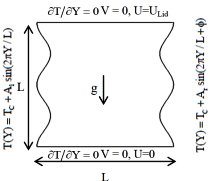Figure 1. Geometry and boundary conditions of cavity

2.1 Mathematical formulation

The governing equations for laminar steady two-dimensional mixed convection, after invoking the Boussinesq approximation and neglecting the viscous dissipation, it can be expressed in the dimensionless form as:

$\frac{\partial \mathrm{U}}{\partial \mathrm{X}}+\frac{\partial \mathrm{V}}{\partial \mathrm{Y}}=0$     (1)

$\mathrm{U} \frac{\partial \mathrm{U}}{\partial \mathrm{X}}+\mathrm{V} \frac{\partial \mathrm{U}}{\partial \mathrm{Y}}=-\frac{\partial \mathrm{P}}{\partial \mathrm{X}}+\frac{1}{\operatorname{Re}}\left(\frac{\partial^{2} \mathrm{U}}{\partial \mathrm{X}^{2}}+\frac{\partial^{2} \mathrm{U}}{\partial \mathrm{Y}^{2}}\right)$     (2)

$\mathrm{U} \frac{\partial \mathrm{V}}{\partial \mathrm{X}}+\mathrm{V} \frac{\partial \mathrm{V}}{\partial \mathrm{Y}}=-\frac{\partial \mathrm{P}}{\partial \mathrm{Y}}+\frac{1}{\operatorname{Re}}\left(\frac{\partial^{2} \mathrm{V}}{\partial \mathrm{X}^{2}}+\frac{\partial^{2} \mathrm{V}}{\partial \mathrm{Y}^{2}}\right)$     (3)

$\mathrm{U} \frac{\partial \theta}{\partial \mathrm{X}}+\mathrm{V} \frac{\partial \theta}{\partial \mathrm{Y}}=\frac{1}{\operatorname{Re} \operatorname{Pr}}\left(\frac{\partial^{2} \theta}{\partial \mathrm{X}^{2}}+\frac{\partial^{2} \theta}{\partial \mathrm{Y}^{2}}\right)$     (4)

In the above equations, the dimensionless variables are defined by:

$\mathrm{X}=\frac{\mathrm{x}}{\mathrm{L}}, \quad \mathrm{Y}=\frac{\mathrm{y}}{\mathrm{L}}, \quad \mathrm{U}=\frac{\mathrm{u}}{\mathrm{U}_{0}}$

$\mathrm{V}=\frac{\mathrm{v}}{\mathrm{U}_{0}}, \mathrm{P}=\frac{\mathrm{p}}{\rho \mathrm{U}_{0}^{2}}, \quad \theta=\frac{\mathrm{T}-\mathrm{T}_{\mathrm{c}}}{\mathrm{T}_{\mathrm{h}}-\mathrm{T}_{\mathrm{c}}}$     (5)

where U and V are the velocity components in the X and Y directions, respectively, θ is the dimensionless temperature and P is the dimensionless pressure. The governing parameters, namely; the Reynolds number Re, Richardson number Ri, Prandtl numbers Pr are defined in the following forms:

\begin{align} & \operatorname{Re}=\frac{{{U}_{0}}L}{\upsilon },\,\,\Pr =\frac{\nu }{\alpha }; \\ & Ri=\frac{Gr}{{{\operatorname{Re}}^{2}}},\,Gr=\frac{g\beta {{L}^{3}}({{T}_{h}}-{{T}_{c}})}{{{\upsilon }^{2}}} \\\end{align}             (6)

The physical dimensionless form of the boundary conditions can be written as:

At the top sliding lid: U=Ulid = 1, V = 0, $\partial \theta / \partial \mathrm{X}=0$

At the bottom wall: U= V = 0, $\partial \theta / \partial \mathrm{X}=0$

At the left vertical wall: U = V = 0, $\theta=\sin (2 \pi \mathrm{Y})$

At the right vertical wall: U = V = 0, $\theta=\varepsilon \sin (2 \pi \mathrm{Y}+\phi)$

Where $\varepsilon=\mathrm{Ar} / \mathrm{Al}$ is the amplitude ratio of the sinusoidal temperature on the right side wall to that on the left side wall, and $\mathrm{A}_{\mathrm{r}}=\mathrm{H} / \mathrm{L}$ is the aspect ratio of the cavity.

The fluid flow structure inside the enclosure is visualized by streamlines, and thus the stream functions ($\Psi$) are defined as:

$\mathrm{U}=\frac{\partial \Psi}{\partial \mathrm{Y}}, \mathrm{V}=-\frac{\partial \Psi}{\partial \mathrm{Y}}$     (7)

The heat transfer coefficient in terms of the local Nusselt number along the left and right-side walls is defined as:

$\mathrm{Nu}_{1}=\left(-\frac{\partial \theta}{\partial \mathrm{N}}\right)_{\mathrm{X}=0}$ and $\mathrm{Nu}_{\mathrm{r}}=\left(-\frac{\partial \theta}{\partial \mathrm{N}}\right)_{\mathrm{X}=1 / \mathrm{Ar}}$     (8)

In the heating half of the side wall, the fluid in the enclosure will gain heat from the side wall and thus Nu > 0, but the fluid will lose heat in the cooling half of the side wall and hence Nu < 0. The total heat transfer rate across the enclosure is the sum of the averaged Nusselt numbers along the heating halves of both vertical side walls, as described by the following average Nusselt number:

$\mathrm{Nu}_{\mathrm{av}}=\int_{\text { heating hal }} \mathrm{Nu}_{1} \mathrm{d} \mathrm{Y}+\int_{\text { heatinghal }} \mathrm{Nu}_{\mathrm{r}} \mathrm{d} \mathrm{Y}$     (9)

2.2 Numerical solution procedure

The numerical method used to solve equations (1) and (4) is the SIMPLE algorithm described by  was used to determine the pressure from the continuity equation, and the discretized equations were solved iteratively in each direction along the uded axes. The deferred Quick scheme of Hayase et al.  is employed to minimize numerical diffusion for the convective terms for both the momentum equations and energy equation. The mass balance for global convergence was taken as 10-6. The computational procedure is validated against the numerical results of [2, 15, 28] for a top heated moving lid and bottom cooled square cavity filled with air (Pr = 0.71). A (128Í128) cartesian mesh is used and computations are done for three different Reynolds and Grashof numbers. The general agreement between the present computation code is verified against the existing results of [2, 15, 28], it is reported in Table 1.

Table 1. Comparison of the computed average Nusselt number with available results in the literature for lid-driven cavity.

 Re Scheme Gr 102 104 106 400 Iwatsu et al.  3.84 3.62 1.22 Sharif  4.05 3.82 1.17 Sivasankaran et al.  4.08 3.84 1.10 Present study 3.87 3.57 1.16 1000 Iwatsu et al.  6.33 6.29 1.77 Sharif  6.55 6.50 1.81 Sivasankaran et al.  6.48 6.47 1.66 Present study 6.52 6.46 1.67

On the other hand, our numerical code is then validated by comparing the numerical results of Deng and Chang , which were reported for natural convection in a rectangular enclosure with sinusoidal temperature distribution on both walls. The comparison was conducted while employing the following dimensionless parameters:$\mathrm{Ra}=10^{3}-10^{5}, \varepsilon=1, \quad \phi=0-\pi$ and $\mathrm{A}_{\mathrm{r}}=1$. An excellent agreement was achieved between results of this present study and the numerical results of Deng and Chang , for both the streamlines and temperature contours inside the cavity as illustrated in Figure 2.

## 39_2.pngFigure 2. Comparison of the streamlines and temperature contours between the present results and those of 

3. Results and Discussion

Several grids have been tested for the case of Re = 400, Ra = 102 on the lid-driven cavity compared with Iwatsu et al. . Table 2 shows the average Nusselt number for the five grids used, at Re = 400. It is clearly seen that there is a little difference between the five results and grid of (128Î128) used in all subsequent calculations. For all the tests investigated, the amplitude of the undulation was A = 0.05.

Table 2. Comparison of the computed average Nusselt number at the hot plate

 Grid 50x50 100x100 150x150 128x128 200x200  Nua 3.988 3.956 3.873 3.720 3.218 3.84

A parametric study was carried out to determine the influence of the wavy wall on the flow fields of air. The Richardson number varying  $0 \leq \mathrm{Ri} \leq 100$ to cover the large range. Most of the investigations are carried out with Pr = 0.71 for different amplitude ratios vary from 0 to 1and phase deviations from 0 to $\pi$ .

3.1 Effect of amplitude ratio

Figures 3 and 4 respectively show, the temperatures and the streamlines distribution of the numerical results for various Richardson number Ri = 0.01-100 and the amplitude ratio is increased from 0 to 1, when the left wall and right wall is uniformly heated. These figures reveal that the isotherms and stream contours change clearly with the increasing of Richardson and amplitude number. Figure 3(a) illustrates the temperature distributions for different Richardson numbers with $\varepsilon=0$ and $\phi=0$. It is noted that the distribution of isotherm is distributed left half of the cavity for $\varepsilon=0$and Ri = 0.01. The same behavior of heat distribution over the cavity appears even at Ri = 1. The isotherms show that the temperature variations are mainly within a thin region near the left sidewall when the Richardson number is increased from 1.0 to 100. This means that a strong thermal boundary layer is formed along the left wall. An increase in the amplitude ratio of 0 to 0.5 is observed from Figure 3 (b). Minimum amount of heat is transferred from both walls for all values of the Richardson number due to non-uniform heating. The effect of increasing the amplitude ratio from 0.5 to 1 provides considerable difference in isotherms and can be seen in Figure 3(c). The heat energy is well distributed from both walls. Strong thermal boundary layers are formed along both walls. Accordingly, heat transfer increases as the amplitude ratio increases.

Figure 4 illustrates streamlines for three different amplitude ratios and the Richardson numbers with $\phi=0$ . The flow pattern containing a single major cell in clockwise direction for Ri = 0.01 exists in Figure 4 (a). The streamlines are concentrated near the moving wall due to the shear force by movement of the top wall. As the Richardson number is increased to 1, the cell is diagonally elongated. It is observed that the shear force is more dominant than the buoyancy in the mixed convection region. This remark is observed in Figure 4 (b-c). When Ri =100 the streamlines indicate that the flow structure is of two symmetric cells. However, in the case where two periodic distributions are imposed on the side walls, both side walls are active and hence the heat transfer is enhanced.

## 3.pngFigure 3. Isotherms for different Richardson number and different $\varepsilon$ with $\phi=0$

## 4.pngFigure 4. Streamlines for different Richardson number and different $\varepsilon$ with $\phi=0$

The variations of the mid-height velocity profile for different amplitude ratios with Pr = 0.71, Ri = 1 and $\phi=0$are illustrated in Figure 5. When the amplitude ratio $\varepsilon=0$, the right wall gets uniform temperature, the velocity of the fluid is increased on increasing the amplitude ratio. Figure 6 (a) and (b) shows the local Nusselt number along the left and right vertical walls respectively for different amplitude ratios at Pr = 0.71, Ri = 1 and $\phi=0$. It reveals that heat transfer rate increases as amplitude ratio increases. Since the amplitude ratio is associated with the right wall only, it does not affect the energy transport on the left wall. It is clearly seen from Figure 6 that the heat transfer rate along the left wall is not affected by changing the amplitude ratio.

## 5.png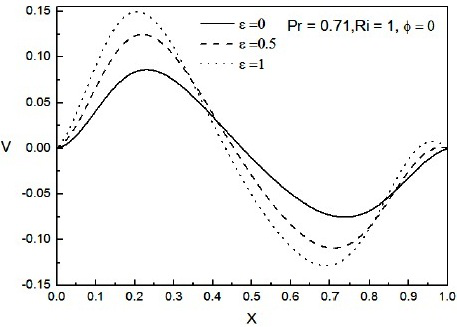Figure 5. Mid-height velocity for different $\varepsilon$

with Ri = 1 and $\phi=0$

## 6.pngFigure 6. Local Nusselt number of the left and right-side walls for different$\varepsilon$ with Ri = 1and $\phi=0$

Figure 7 displays the average Nusselt number versus the Richardson number for different amplitude ratios and$\phi=0$. We note that the shape of the variation of the average Nusselt number is gradually increased as the Richardson number is increased from 0.01 to 100. It is observed from the figure that the average Nusselt number is gradually increased, and decreased as the Richardson number Ri £ 10. For 0 £ Ri£102 the heat transfer rate for $\varepsilon=1$is higher than other two cases of $\varepsilon$ ($\varepsilon=0$and 0.5). The difference in the heat transfer rate among different values of e is negligible in the forced convection dominated regime.

## 7.png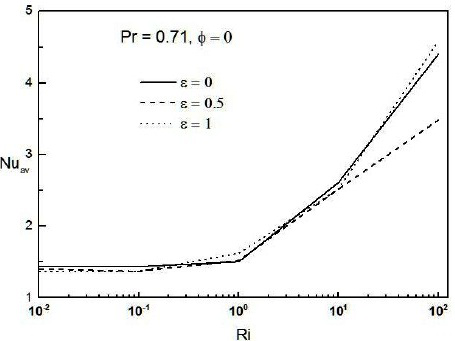Figure 7. Average Nuss   elt number versus Ri for different amplitude ratio with $\phi=0$

3.2 Effect of phase deviation

The variations of the mid-height velocity profile for different various phase deviations $\Phi$ with Pr = 0.71, Ri = 1 and $\varepsilon=1$ are shown in Figure 8. It is found that the changes in phase deviation affect the velocity of the fluid. When phase deviation values are changed from 0 to $\pi$, the heating and cooling zones are placed top and bottom of the left wall of the cavity, respectively. As heating zone is moved upward on increasing$\phi$, the velocity of fluid is first increased and then decreased.

## 8.png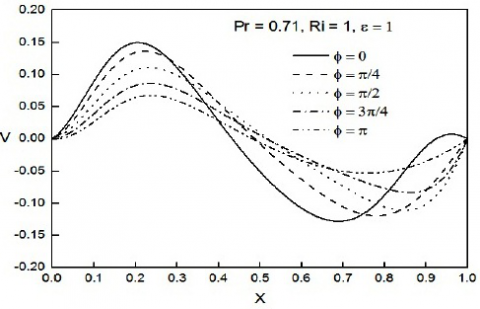Figure 8. Mid-height velocity profiles for different $\phi$with Ri = 1 and $\varepsilon=1$

Figure 9 (a) and (b) illustrates the effect of the phase deviation on local Nusselt number along both left and right sidewalls. It is found that the local Nusselt number along the right wall is significantly affected by changing the phase deviation parameter. The change in phase deviation is slightly affected by the local Nusselt number distribution at the middle of the heating and cooling zones of the left sidewall.

## 9.png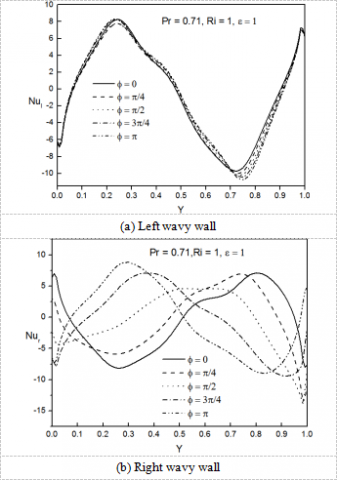Figure 9. Local Nusselt number for different $\phi$ with Ri = 1 and $\varepsilon=1$

## 10.png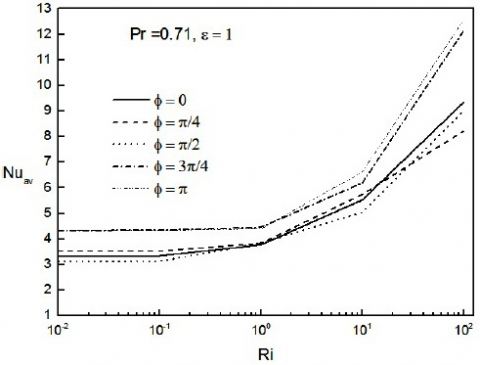Figure 10. Average Nusselt number for different phase deviations with $\varepsilon=1$

The variation of the average Nusselt number across the cavity in terms of Richardson number at various phase deviations $\phi$ of the temperature profiles between the left and right-side walls, and the other parameters are kept constant at Pr = 0.71 and $\varepsilon=1$ as seen in Figure 10. It is found that the average Nusselt number is always increased as the Richardson number increases for each phase deviation $\phi$. It is observed from the figures that the heat transfer rate is enhanced for $\phi=\pi$.

The isotherms and streamlines for different Richardson number and different phase deviation are analyzed in Figures 11 and 12 respectively. As it can be seen from the Figures 11 (a - e), that both walls are divided into two zones, namely, the heating zone and the cooling zone due to sinusoidal heating temperature. The zones on the left wall are fixed while the zones on right wall are moving on increasing the phase deviation. If $\phi=0$, both walls are having the same temperature distributions from bottom to top. The isotherms show that the thermal penetration becomes stronger for all values of phase deviation. In particular thermal boundary layers occur along both walls at Ri = 100. High heat transfer is observed at both values of the phase deviation, $\phi=3 \pi / 4$and $\pi$ . Figure 12 (a - e) indicate the effect of the different phase values on fluid flow when amplitude ratio is a fixed constant. When the Richardson number increases, the streamlines show that two symmetric cells are formed in the upper and lower halves of the cavity for all the phase deviation tested. For $\phi=0$ and Ri = 100 a four-cellular flow structure formed inside the cavity. Among these four cells, cells near the right wall are bigger than the cells near the left wall. This multicellular structure enhances the heat transfer inside the cavity.

3.3 Effect of the Richardson number

Figure 13 illustrates the variations of the mid-height velocity profile for different Richardson number values with Pr = 0.71, $\phi=0$  and $\varepsilon=0.5$ .The velocity of fluid is increases as the Richardson number is increased from 0.01 to 1. The bi-directional velocity profile clearly shows the multi-cellular flow structure for Ri = 100. As convection mode is changed from forced convection to natural convection, the fluid velocity is raised.

## 11.png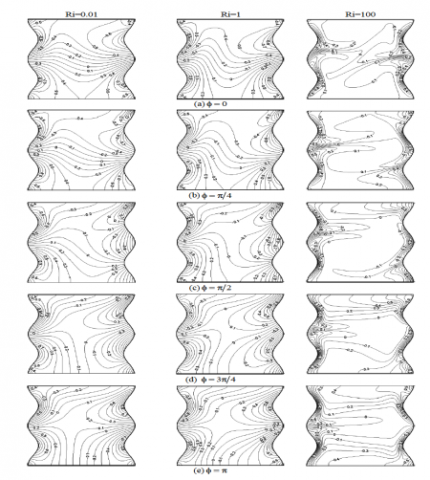Figure 11. Isotherms for different Richardson number and different phase deviation with e = 1

## 12.pngFigure 12. Streamlines for different Richardson number and different phase deviation with e = 1

## 13.png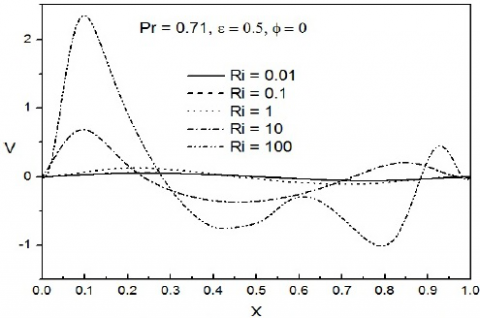Figure 13. Mid-height velocity profiles for different Richardson numbers

The effect of Richardson number on local Nusselt number along both left and right sidewalls with Pr =0.71, $\varepsilon=0.5$ and $\phi=0$ is shown in Figure 14. However, it may be noted that  the diagonally symmetric flow structure, the local Nusselt number along the right side wall is reversed and thus omitted. The curves are approximately of sinusoidal shape like the thermal boundary, which indicates that the local heat transfer is directly affected by the temperature distribution on the surface. The maximal local Nusselt number on the lower heating half is larger than the minimal value on the upper half, i.e., more heat is transferred from the lower heating half than from the upper half of the left side wall. This is consistent with the flow structure, as a larger cell is formed along the lower heating half than along the upper cooling half.

## 14.png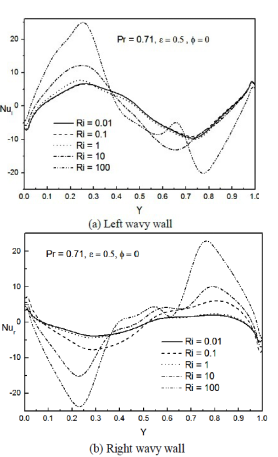Figure 14. Local Nusselt number for different Richardson numbers

3.4 Average Nusselt number

## 15.png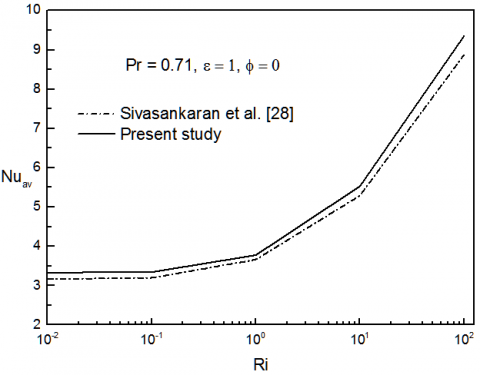Figure 15. Variation of the average Nusselt number with Richardson number for Pr =0.71, $\varepsilon=1$ and f =0

A comparison between the results of this present study and those of Sivasankaran et al.  for the variation of average Nusselt number versus the Richardson number for $\varepsilon=1$, $\phi=0$ and Pr = 0.71 is shown in Figure 15. It can be seen that the average Nusselt number increased gradually as the Richardson number is increased from 0.01 to 100. We note that the average Nusselt number obtained in the present study increase slightly higher than that of , this increase is due to the wavy wall. This comparison shows a good agreement with an average error less than 4.38 %.

4. Conclusions

Mixed convection flow and heat transfer in a new proposed complicated cavity in this paper with non-uniform heating on both sidewalls have been analyzed numerically. The effect of Richardson numbers, amplitude ratios and phase deviation in heat and mass transfer have been studied in detail. The main conclusions can be summarized as follows:

- The heat transfer is increased as the amplitude ratio $\varepsilon$ increases from 0 to 1. This means that the non-uniformly sinusoidal temperature distribution on side wall is beneficial for improving heat transfer as compared to the case where the wall is kept at uniform temperature.

- High temperature gradients are observed near both  hot sidewalls, which causes an increase in the convection heat transfer. While, in the other regions of the cavity, the temperature differences are slight and as a result, the temperature gradients are small due to the important effect of the lid-driven.

- The two wavy sidewalls with a non-uniform heating have a very important effect on heat transfer compared with plane walls.

- It is found that the average Nusselt number always increases as the Richardson number increases for each phase deviation $\phi$ and the heat transfer rate is enhanced for $\phi=\pi$.

- Results obtained in this present study were also confronted with those of [2, 15, 26, 28].  This comparison shows very good agreement.

Finally, it should be noted that the study of the new proposed cavity presents a very interesting alternative due to the wavy walls with a non-uniform temperature distribution. It becomes competitive opposite to other simple cavities with a constant temperature distribution.

Acknowledgment

The helpful comments by the reviewers are gratefully acknowledged by the authors.

Nomenclature
 A amplitude, m Cp specific heat, J.kg-1. K-1 g gravitational acceleration, m. s-2 p pressure, Pa k thermal conductivity, W.m-1. K-1 L cavity height/width, m T temperature, K P dimensionless pressure Pr Prandtl number Re Reynolds number Ri Richardson number Gr Grashof number NuL Nusselt local number Nuav average Nusselt number U, V dimensionless velocity components along X and Y, respectively ULid sliding left wall velocity, m. s-1 x, y cartesian coordinates, m X, Y dimensionless coordinate Greek symbols $\alpha$ thermal diffusivity, m2. s-1 $\beta$ thermal expansion coefficient, K-1 $\varepsilon$ amplitude ratio $\theta$ dimensionless temperature $\rho$ density, Kg. m-3 $v$ kinematic viscosity, m2. s-1 $\Psi$ stream function $\Psi$ dimensionless stream function $\phi$ phase deviation Subscripts l left wall r right wall 0 reference state
References

 Iwatsu R., Hyun J.M., Kuwahara K. (1992). Numerical simulation of flows driven by a torsionally oscillating lid in a square cavity, J. Fluids Eng., Vol. 114, pp. 143-151. DOI: 10.1115/1.2910008

 Iwatsu R., Hyun J.M., Kuwahara K. (1993). Mixed convection in a driven cavity with a stable vertical temperature gradient, Intl. J. Heat Mass Transfer, Vol. 36, pp. 1601-1608. DOI: 10.1016/S0017-9310(05)80069-9

 Iwatsu R., Hyun J.M. (1995). Three-dimensional driven cavity flows with a vertical temperature gradient, Intl. J. Heat Mass Transfer, Vol. 38, pp. 3319-3328. DOI: 10.1016/0017-9310(95)00080-S

 Gebhart B., Jaluria Y., Mahajan R.L., Sammakia B. (1988). Buoyancy Induced Flows and Transport, Hemisphere, New York, pp. 699-723.

 Hasnaoui M., Bilgen E., Vasseur P. (1990). Natural convection above an array of open cavities heated from below, AIAA J. Thermophys. Heat Transfer, Vol. 6, pp. 255-264. DOI: 10.1080/10407789008944803

 Lee S.C., Chen C.K. (1996). Finite element solutions of laminar and turbulent mixed convection in a driven cavity, Int. J. Numer. Methods Fluids, Vol. 23 pp. 47-64.

 Moraga N., López S. (2004). Numerical simulation of three-dimensional mixed convection in an air-cooled cavity, Numerical Heat Transfer, Part A, Vol. 45, No. 8, pp. 811-824. DOI:  10.1080/10407780490250409

 Wang C.C., Chen C.K. (2002). Forced convection in a wavy-wall channel, Intl. J. Heat Mass Transfer, Vol. 45, pp. 2587-2595. DOI: 10.1016/S0017-9310(01)00335-0

 Al-Amiri A., Khanafer K., Bull J., Ioan P. (2007). Effect of sinusoidal wavy bottom surface on mixed convection heat transfer in a lid-driven cavity, Intl. J. Heat Mass Transfer, Vol. 9, No. 50, pp. 1771-1780. DOI: 10.1016/j.ijheatmasstransfer.2006.10.008

 Zeghbid I., Bessaih R. (2015). mixed convection in lid-driven cavities filled with a nanofluid, International Journal of Heat and Technology, Vol. 33, No. 4, pp. 77-84. DOI: 10.18280/ijht.330410

 Khan W.A., Gorla R.S.R. (2010). Mixed convection of power-law fluids along a vertical wedge with convective boundary condition in a porous medium, Journal of Mechanical Science and Technology, Vol. 24, No. 9, pp. 1313-1325. DOI: 10.1007/s12206-010-0624-9

 Adjlout L., Imine O., Azzi A., Belkadi M. (2002). Laminar natural convection in an inclined cavity with a wavy wall, Intl. J. Heat Mass Transfer, Vol. 45, No. 10, pp. 2141-2152. DOI: 10.1016/S0017-9310(01)00304-0

 Aich W., Hajri I., Omri A. (2011). Numerical analysis of natural convection in a prismatic enclusure, Thermal Science, Vol. 15, No. 2, pp. 437-446. DOI: 10.2298/TSCI1102437A

 Ambethka V., Kushawaha D. (2017). Numerical simulations of fluid flow and heat transfer in a four-sided lid-driven rectangular domain, I International Journal of Heat and Technology, Vol. 35, No. 2, pp. 235-242. DOI: 18280/ijht.350207

 Sharif M.A.R. (2007). Laminar mixed convection in shallow inclined driven cavities with hot moving lid on top and cooled from bottom, Applied Thermal Engineering, Vol. 27, pp. 1036-1042. DOI: 10.1016/j.applthermaleng.2006.07.035

 Boutara A., Ragui.K., Benkahla Y.K. (2017). Free convection enhancement within a nanofluid filled enclosure with square heaters, International Journal of Heat and Technology, Vol. 35, No. 3, pp. 447-4458. DOI: 10.18280/ijht.350302

 Kumar B.V.R. (2000). A study of free convection induced by a vertical wavy surface with heat flux in a porous enclosure, Numerical Heat Transfer, Vol. 37, No. 5, pp. 493-510. DOI: 10.1080/104077800274181

 Yao L.S. (1983). Natural convection along a vertical wavy surface, J. Heat Transfer, Vol. 105, pp. 465-468.

DOI: 10.1115/1.3245608

 Mekroussi S., Driss N., Bouzit M., Chemloul N.S. (2013). Analysis of mixed convection in an inclined lid-driven cavity with a wavy wall, Journal of Mechanical Science and Technology, Vol. 27, No. 7, pp. 2181-2190. DOI: 10.1007/s12206-013-0533-9

 Lorenzini G., Machado B.S., Isoldi L.A., Dos Santos E.D., Rocha L.A.O. (2016). Constructal design of rectangular fin intruded into mixed convective lid-driven cavity flows, ASME Journal of Heat Transfer, Vol. 138, No. 10, pp. 102501-1/102501-12. DOI: 10.1115/1.4033378

 Hamdi M., Meziani B., Sadaoui D. (2017). Numerical study of mixed convection and flow pattern in various across-shape concave enclosures, International Journal of Heat and Technology, Vol. 35, No. 3, pp. 273-278. DOI: 10.18280/ijht.350207

 Ambethka V., Kumar M. (2017). Numerical solutions of 2-D unsteady incompressible flow with heat transfer in a driven square cavity using streamfunction-vorticity formulation, International Journal of Heat and Technology, Vol. 35, No. 3, pp. 459-473. DOI: 10.18280/ijht.350303

 Oztop H., Varol A. (2009). Combined convection in inclined porous lid-driven enclosures with sinusoidal thermal boundary condition on one wall, Progress in Computational Fluid Dynamics, Vol. 9, pp. 127-131. DOI: 10.1504/PCFD.2009.023356

 Bae K.Y., Jeong H.M., Chung H.S. (2004). Study on natural convection in a rectangular enclosure with a heating source, KSME International Journal, Vol. 18, No. 2, pp. 294-301. DOI: 10.1007/BF03184739

 Dalal A., Das M.K. (2005). Laminar natural convection in an inclined complicated cavity with spatially variable wall temperature, Intl. J. Heat Mass Transfer, Vol. 48, No. 18, pp. 3833-3854. DOI: 10.1016/j.ijheatmasstransfer.2004.07.050

 Deng H.Q., Chang. J.J. (2008). Natural convection in a rectangular enclosure with sinusoidal temperature distributions on both side walls, Numerical Heat Transfer, Part A, Vol. 54, pp. 507-524. DOI: 10.1080/01457630802186080

 Natarajan E., Basak T., Roy S. (2008).  Natural convection flows in a trapezoidal enclosure with uniform and non-uniform heating of bottom wall, Int J Heat Mass Transfer, Vol. 51, pp. 747-756. DOI: 10.1016/j.ijheatmasstransfer.2007.04.027

 Sivasankaran S., Sivakumar V., Prakash P. (2010).  Numerical study on mixed convection in a lid-driven cavity with non-uniform heating on both side walls, Int. J. Heat Mass Transfer, Vol. 53, pp. 4304–4315. DOI: 10.1016/j.ijheatmasstransfer.2010.05.059

 Sivasankaran S., Pan K.L. (2012). Numerical simulation on mixed convection in a porous lid-driven cavity with non-uniform heating on both side wall, Numerical Heat Transfer, Part A 61, pp. 101-121. DOI: 10.1080/10407782.2011.643741

 Abbasian A.A.A., Mazrouei S., Mahmoodi M., Ardeshiri A., Aliakbari M. (2012).  Numerical study of mixed convection flow in a lid-driven cavity with sinusoidal heating on sidewalls using nanofluid, Superlattices Microstruct, Vol. 51, pp. 893-911. DOI: 10.1016/j.spmi.2012.02.015

 Patankar S.V. (1980). Numerical Heat Transfer and Fluid Flow, Hemisphere/McGraw-Hill, Washington D.C.

 Hayase T., Humphrey J.C., Greif R. (1992). A consistently formulated quick scheme for fast and stable convergence using finite-volume iterative calculation procedures, J. Comput. Phys., Vol. 98, pp. 118-180. DOI: 10.1016/0021-9991(92)90177-Z